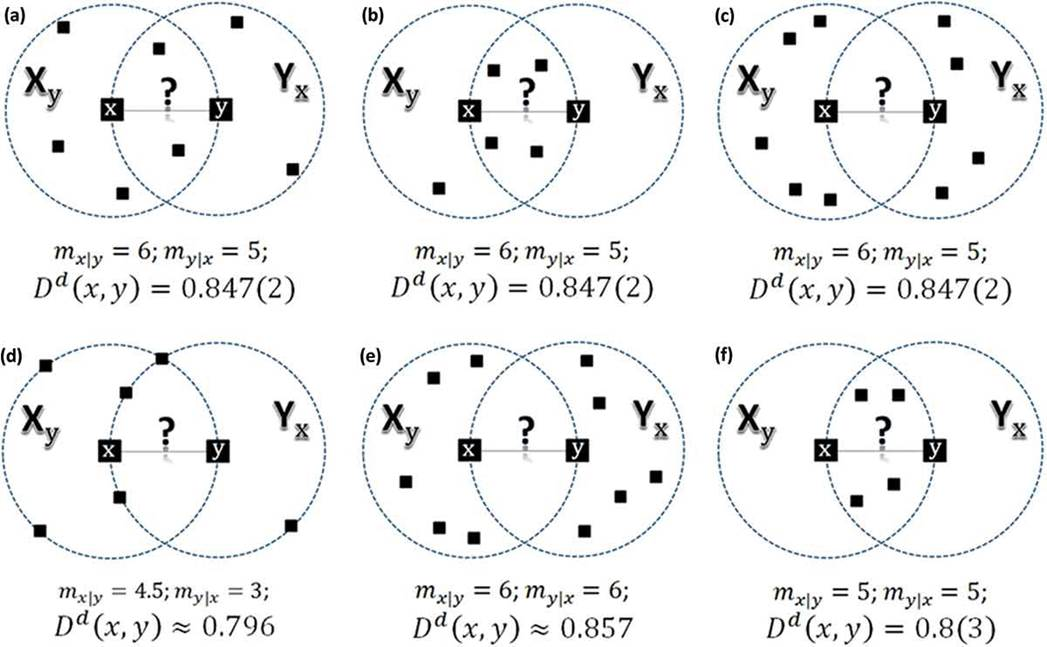Reference:   Terziyan V., Social Distance Metric: From Coordinates to Neighborhoods, International Journal of Geographical Information Science, 31 (12), 2401-2426, Taylor & Francis, 2017. (doi: 10.1080/13658816.2017.1367796)

For any two points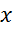and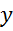in some data space with metric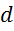, we define the “mutual social ranking” function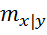as follows: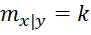, if pointis the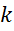-th nearest neighbor of the pointin metric; and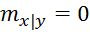, if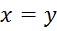. Similarly: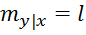, if pointis the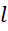-th nearest neighbor of the pointin metric. It is evident thatand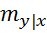are not necessarily equal (see the example of the mutual social ranking asymmetry in the figure below where the pointfor the pointis the eighth closest one while the pointfor the pointis the fifth closest one).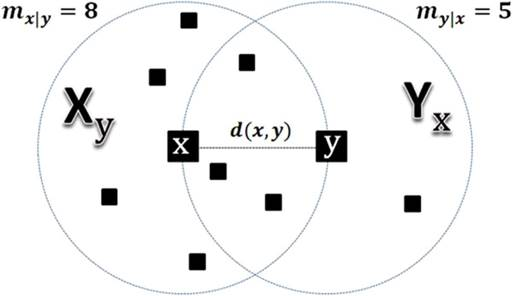Special case (“rule of tie”): If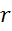points (including point) pretend to be the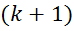-th nearest neighbor of point, then: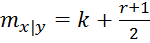.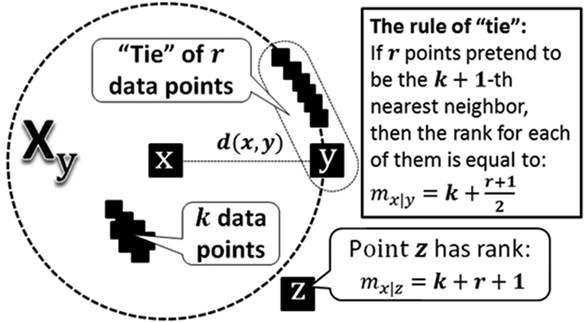Havinganddue to the use of some metric, and choosing, we can compute the Social Distance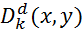betweenandas follows:

I.                First we averageandin a special way. We compute the Lehmer mean ofand, which is: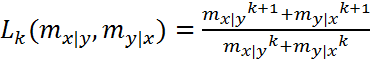.

Notice that this averaging function includes most of famous means depending on. E.g., it is equals to Arithmetic mean for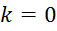, and to Contraharmonic mean for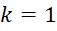See more cases and details in the referred article.

II.              Using the Lehmer mean above, we compute the Social Distance as follows: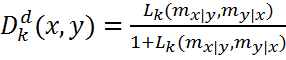.

and we have proven it (and several modifications of it) to be a metric suitable for many intelligent data processing tasks (classification, clustering, etc.) See details in the article.

See examples of the Social Distance computing for different neighborhoods in 2D space for the same pair of data pointsand: cases (a)–(c) have different configuration resulting to the same distance; (d) case with some data points at the border of the neighborhood (ties); (e) and (f) cases with symmetric neighborhoods.